Math Olympiad Test: Surface Area and Volume- 1

# Math Olympiad Test: Surface Area and Volume- 1

Test Description

## 10 Questions MCQ Test Mathematics Olympiad for Class 9 | Math Olympiad Test: Surface Area and Volume- 1

Math Olympiad Test: Surface Area and Volume- 1 for Class 9 2022 is part of Mathematics Olympiad for Class 9 preparation. The Math Olympiad Test: Surface Area and Volume- 1 questions and answers have been prepared according to the Class 9 exam syllabus.The Math Olympiad Test: Surface Area and Volume- 1 MCQs are made for Class 9 2022 Exam. Find important definitions, questions, notes, meanings, examples, exercises, MCQs and online tests for Math Olympiad Test: Surface Area and Volume- 1 below.
Solutions of Math Olympiad Test: Surface Area and Volume- 1 questions in English are available as part of our Mathematics Olympiad for Class 9 for Class 9 & Math Olympiad Test: Surface Area and Volume- 1 solutions in Hindi for Mathematics Olympiad for Class 9 course. Download more important topics, notes, lectures and mock test series for Class 9 Exam by signing up for free. Attempt Math Olympiad Test: Surface Area and Volume- 1 | 10 questions in 10 minutes | Mock test for Class 9 preparation | Free important questions MCQ to study Mathematics Olympiad for Class 9 for Class 9 Exam | Download free PDF with solutions
 1 Crore+ students have signed up on EduRev. Have you?
Math Olympiad Test: Surface Area and Volume- 1 - Question 1

### The length of cold storage is double its breadth. Its height is 3 meters. If the area of four walls including doors is 108 m2, what is its volume?

Detailed Solution for Math Olympiad Test: Surface Area and Volume- 1 - Question 1

Let l = 2b, h = 3m
Area of four walls = 108
2 (l + b) h = 108
⇒ 2 (2b + b) 3 = 108
⇒ 3b = 108/6 = 18
⇒ b = 18/3 = 6
∴ l = 2b = 2 × 6 = 12
∴ Volume of cold storage
= lbh = 12 × 6 × 3 = 216 m3

Math Olympiad Test: Surface Area and Volume- 1 - Question 2

### The paint in a certain container is sufficient to paint an area of 9.375 m2. How many bricks of dimension 22.5 cm × 10 cm × 7.5 cm can be painted out of this container?

Detailed Solution for Math Olympiad Test: Surface Area and Volume- 1 - Question 2

No. of bricks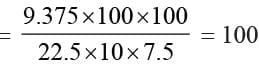Math Olympiad Test: Surface Area and Volume- 1 - Question 3

### A solid cube is cut into two cuboids of equal volumes. Find the ratio of total surface area of given cube and that of one cuboid.

Detailed Solution for Math Olympiad Test: Surface Area and Volume- 1 - Question 3

Volume of cuboid = a3/2 = Ibh
∴ Surface area of each cuboid
= 2 (lb + bh + lh)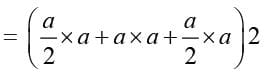= 2 (2a2) = 4a2
Total surface area of cube = 6a2
∴ Required ratio = 6a2 : 4a2 = 3 : 2

Math Olympiad Test: Surface Area and Volume- 1 - Question 4

The diameter of the roller 1.5 m long is 84 cm. If it takes 100 revolutions to level a playground, what is the cost of leveling the playground at the rate of 50 paise per square meter?

Detailed Solution for Math Olympiad Test: Surface Area and Volume- 1 - Question 4

The curved surface area of the roller = 2πrh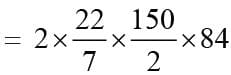= 44 × 150 × 6
Area covered by roller in 100 revolutions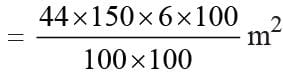Cost of leveling the playground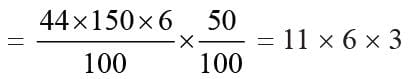= ₹ 198

Math Olympiad Test: Surface Area and Volume- 1 - Question 5

The volume of a cylinder is 448 π cm3 and height 7 cm. What is the lateral surface area of the cylinder?

Detailed Solution for Math Olympiad Test: Surface Area and Volume- 1 - Question 5

Volume of the cylinder = 448π
⇒ πr2h = 448π
⇒ r2h = 448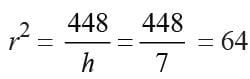⇒ r = 8 cm
Lateral surface area = 2πrh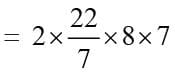= 22 × 16 = 352 cm2

Math Olympiad Test: Surface Area and Volume- 1 - Question 6

At a meal, a stall keeper in one of the food stalls has a large cylindrical vessel of base radius 15cm filled up to a height of 32 cm with fruit juice. The juice is filled in small cylindrical glasses of radius 3cm up to a height of 8cm. how many glasses will be filled by selling the juice completely?

Detailed Solution for Math Olympiad Test: Surface Area and Volume- 1 - Question 6

Let the number of glasses be n
∴ Total volume in the vessel = volume of juice in glasses
⇒ πR2H = n × πr2h
⇒ R2H = nr2h
⇒ (15)2 × 32 = n × (3)2 × 8
⇒ n = 5 × 5 × 4 = 100

Math Olympiad Test: Surface Area and Volume- 1 - Question 7

How many meters of cloth of 5 m width will be required to make a conical tent. The radius of whose base is 7 m and heights is 24 m?

Detailed Solution for Math Olympiad Test: Surface Area and Volume- 1 - Question 7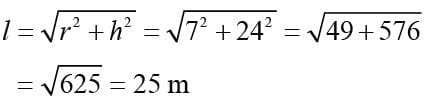Curved surface = πrl = 22/7 × 7 × 25 = 550 m2
Length of canvas used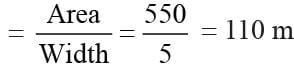Math Olympiad Test: Surface Area and Volume- 1 - Question 8

The diameter of the moon is approximately 1/4th of the diameter of the earth. What fraction of the volume of earth is the volume of the moon?

Detailed Solution for Math Olympiad Test: Surface Area and Volume- 1 - Question 8

Let the diameter of the earth be x m
∴ Diameter of moon = x/4 m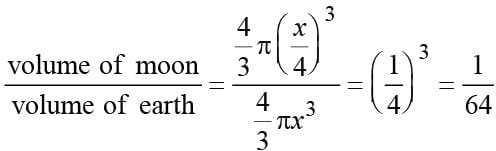Math Olympiad Test: Surface Area and Volume- 1 - Question 9

Water flows in a tank 150 m × 100 m at the base through a pipe whose cross-section is 2 dm by 1.5 dm at the speed of 15 km per hour. At what time will the water be 3 meters deep?

Detailed Solution for Math Olympiad Test: Surface Area and Volume- 1 - Question 9

The volume of water in the tank
= 150 × 100 × 3 = 45000 m3
Area of a cross-section of the pipe
= 2dm × 1.5dm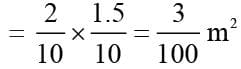Let the time taken be t hours.
The volume of water that flows in a tank in t hours.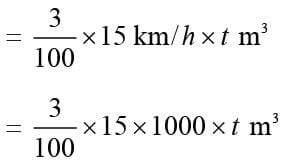= 450 t m3
⇒ 450t = 45000
⇒ t = 45000/450 = 100 hours

Math Olympiad Test: Surface Area and Volume- 1 - Question 10

In a shower, 5cm of rainfalls. What is the volume of water that falls on 2 hectares of the ground?

Detailed Solution for Math Olympiad Test: Surface Area and Volume- 1 - Question 10

Volume of water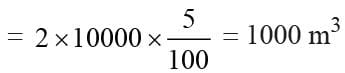## Mathematics Olympiad for Class 9

1 videos|43 tests
 Use Code STAYHOME200 and get INR 200 additional OFF Use Coupon Code
Information about Math Olympiad Test: Surface Area and Volume- 1 Page
In this test you can find the Exam questions for Math Olympiad Test: Surface Area and Volume- 1 solved & explained in the simplest way possible. Besides giving Questions and answers for Math Olympiad Test: Surface Area and Volume- 1, EduRev gives you an ample number of Online tests for practice

## Mathematics Olympiad for Class 9

1 videos|43 tests